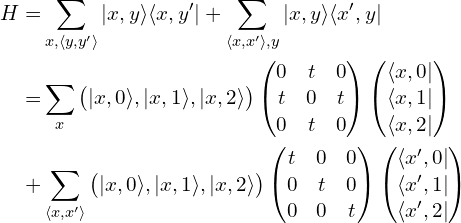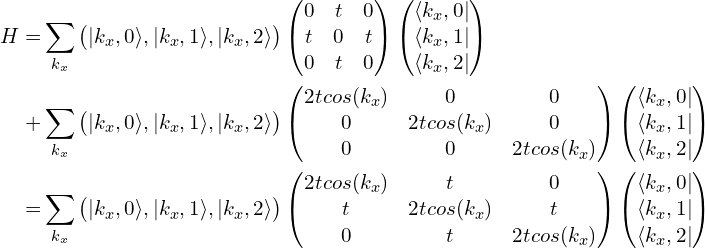# 准一维方格子能带图（附Python代码）"""
This code is supported by the website: https://www.guanjihuan.com
The newest version of this code is on the web page: https://www.guanjihuan.com/archives/3895
"""

import numpy as np
import matplotlib.pyplot as plt
from math import *
import cmath
import functools

def hamiltonian(k, N, t):  # 准一维方格子哈密顿量
# 初始化为零矩阵
h00 = np.zeros((N, N), dtype=complex)
h01 = np.zeros((N, N), dtype=complex)
for i in range(N-1):   # 原胞内的跃迁h00
h00[i, i+1] = t
h00[i+1, i] = t
for i in range(N):   # 原胞间的跃迁h01
h01[i, i] = t
matrix = h00 + h01*cmath.exp(1j*k) + h01.transpose().conj()*cmath.exp(-1j*k)
return matrix

def main():
H_k = functools.partial(hamiltonian, N=10, t=1)
k = np.linspace(-pi, pi, 300)
plot_bands_one_dimension(k, H_k)

def plot_bands_one_dimension(k, hamiltonian):
dim = hamiltonian(0).shape
dim_k = k.shape
eigenvalue_k = np.zeros((dim_k, dim))
i0 = 0
for k0 in k:
matrix0 = hamiltonian(k0)
eigenvalue, eigenvector = np.linalg.eig(matrix0)
eigenvalue_k[i0, :] = np.sort(np.real(eigenvalue[:]))
i0 += 1
for dim0 in range(dim):
plt.plot(k, eigenvalue_k[:, dim0], '-k')
plt.show()

if __name__ == '__main__':
main()


【未经同意请勿转载。引用请注明出处：https://www.guanjihuan.com

3,877 次浏览

## 6 thoughts on “准一维方格子能带图（附Python代码）”

1.匿名说道：

楼主您好，请问假如原胞内的跃迁不是一个数而是一个矩阵，那么构建哈密顿量的时候怎么赋值呢？

1.guanjihuan说道：
2.匿名说道：

问一下博主，这个方格子示意图水平方向是无限长的是吗？

1.guanjihuan说道：

是的

3.匿名说道：

大神，想请教一个问题，对于两个方向都是有限的模型，比如你那篇方格子的哈密顿量，哈密顿量里面都是固定的跃迁系数，与k无关，如何画出他们的能带结构呢？

1.guanjihuan说道：

能带是要求在某个方向是周期性的，这样才可以傅里叶变换，才有动量k。如果体系没有周期性，当然也可以把整个有限宽度和高度的体系当成元胞，在某个方向上周期性重复，这样也能画出能带，也能体现出该体系的性质。但这种情况能带的结构会比较乱，这是因为元胞内包含的自由度比较高，对应的带也就比较多了。